Search

Proving that Two Functions are
Inverses of Each Other
(page 7 of 7)

Sections: Definition / Inverting a graph, Is the inverse a function?, Finding inverses, Proving inversesI have shown how to draw an inverse if you're given the graph, and how to find an inverse if you're given the formula. But suppose you are given two functions and told to verify (to check) that they are inverses of each other. How would you do that? First, you would need to note that drawing the graphs is not a "proof". To emphasize that a picture isn't proof, the instructions will often tell you to "verify algebraically" that the functions are inverses. How do you do that?

If you think back to the definition of an inverse, the point of the inverse is that it's backwards from what you started with; it takes you back to where you started from. For instance, if the point (1, 3) is on the graph of the function, then the point (3, 1) is on the graph of the inverse. That is, if you start with x = 1, you will go to y = 3; then you plug this into the inverse, and you'll go right back to x = 1, where you started from.

It is this property that you use to prove (or disprove) that functions are inverses of each other. You will compose the functions (that is, plug x into one function, plug that function into the inverse function, and then simplify) and verify that you end up with just "x". Here's what it looks like:

• Determine algebraically whether  f (x) = 3x  2 and g(x) = (x + 2)/3 are inverses of each other.  Copyright © Elizabeth Stapel 2000-2011 All Rights Reserved

I will plug the formula for g(x) into every instance of "x" in the formula for f (x):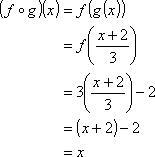Now I will plug  the formula for f (x) into every instance of "x" in the formula for g(x) :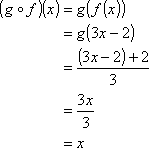Both ways, I ended up with just "x", so  f (x) and g(x) are inverses of each other.

• Determine algebraically whether  f (x) = 3x  2 and g(x) = (1/3)x + 2 are inverses of each other.

I'll plug the formula for g(x) into every instance of "x" in the formula for f (x):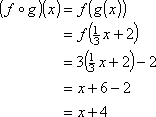I didn't end up with just "x", so  f (x) and g(x) are not inverses of each other.

Once you've found one composition that doesn't work, you're done. You don't have to show that the composition doesn't work the other way, either.

A close examination of this last example above points out something that can cause problems for some students. Since the inverse "undoes" whatever the original function did to x, the instinct is to create an "inverse" by applying reverse operations. In this case, since  f (x) multiplied x by 3 and then subtracted 2 from the result, the instinct is to think that the inverse would be to divide x by 3 and then to add 2 to the result. But as you saw above, this is not correct. Comparing this example with the previous example, you can see that the reversed operations were correct, but that they also need to be applied in reverse order. That is, since f (x) first multiplied x by 3 and then subtracted off 2, the inverse first adds the 2 back on, and then divides the 3 back off.

Also, in the second example, as soon as I did not end up with "x", I knew the functions were not inverses. I had done the composition ( f o g)(x) and had come up with something other than "x", so I didn't bother checking (g o f )(x). In the first example, however, I checked ( f o g)(x) and came up with "x", and then I also checked (g o f )(x), too. Why? Here's an example of why:

• Determine algebraically whether  f (x) = x2and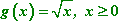are inverses of each other.

First, I'll plug g(x) into  f (x):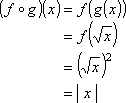Since I started by plugging x into g(x), then I started with non-negative x-values. Since the absolute value of zero is zero and the absolute value of a positive number is just itself, then, in this case, I can simplify | x | as just "x". Then I have ( f o g)(x) x.

Where did the absolute-value bars come from? The square root of something squared is the technical definition of the absolute value: the square of the value will always be positive, as will the square root, so taking the square root of something squared always returns the positive of the original number. In this case, the domain of g(x) was defined as non-negative, so the absolute-value bars could be dropped above. But this isn't always the case:

Looking good so far. Now I'll plug  f (x) into g(x):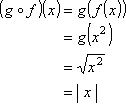Hmm... Since I started by plugging x into  f (x), then I was starting with any value of x. In particular, the value of x might have been negative. Since I don't know if x is negative or positive, then I can't remove the absolute-value bars on the final answer, and I'm stuck with an answer of "(g o f )(x) = | x |". So (g o f )(x) does not simplify to x.

The answer is:  g(x) and  f (x) are not inverses of each other.

This is why you need to check both ways: sometimes there are fussy technical considerations, usually involving square roots, that force the composition not to work, because the domains and ranges of the two functions aren't compatible. In this case, if f (x) had been restricted to non-negative x, then the functions would have been inverses. In general, though, if one composition gives you just "x", then the other one will, too, especially if you're not dealing with restricted domains. But you should remember to do both compositions on tests and such, in order to get full credit.

<< Previous  Top  |  1 | 2 | 3 | 4 | 5 | 6 | 7  |  Return to Index

 Cite this article as: Stapel, Elizabeth. "Proving that Two Functions are Inverses of Each Other."     Purplemath. Available from https://www.purplemath.com/modules/invrsfcn7.htm.     Accessed [Date] [Month] 2016

Study Skills Survey

Tutoring from Purplemath
Find a local math tutor